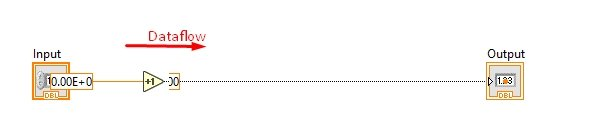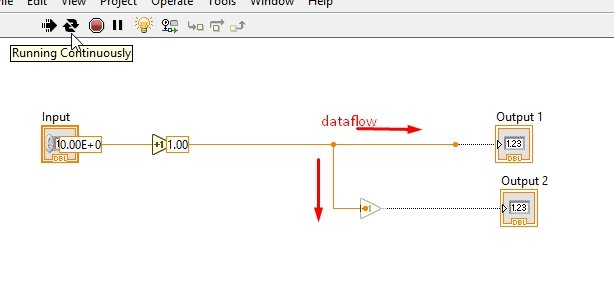# Data Flow in LabView : tutorial 20

In this tutorial you fill learn the dataflow of LabView. One might be wondering what dataflow is?? Data flow is the flow of data during runtime. How the block diagram is executed when we run the VI i.e. what is the order of execution is it from left to right or top to bottom (as in text based programming languages). At the start an explanatory introduction of dataflow in LabView is given. After that a VI is designed with the help of which data flow is explained in detail. At the end of the tutorial you are provided with an exercise to do it by yourself, and in the next tutorials I will assume that you have done those exercises and I will not explain the concept regarding them.

### Introduction to Data Flow in labview

In computer science the term data flow is used extensively and is based on the idea of disconnecting computational actors into stages that can execute concurrently. Streaming process is another term that can be used concurrently with dataflow.

Dataflow or streaming process languages have been subdivided into several forms. We can also define the architecture of the hardware of dataflow as an alternative to Von Neumann architecture. Reactive programming with spreadsheets is the most obvious example of data-flow programming. The entered values by a user are instantly transmitted to the next logical variable or formula for calculation.

In LabView dataflow is consider as one of the very important terms. A node present in a block diagram will execute only when data is present at all of its input as you will see later in this tutorial. After the execution of a node the output data of the node is transmitted to the next node of the dataflow path. The execution order of the VIs and function on the block diagram is determined by the movement of data through the nodes.

### Examples of Data Flow in LabView

Figure 1: Increment placement

Figure 2: Block diagram

Figure 3: Highlights

• Enabling this button will allow you to see the flow of data through the wires in respective block diagram and will help you see easily the execution flow in you VI. Now, run the program using run button orb by pressing <Ctrl+R> and see the flow of the data.
• The nodes or wires in which data is not flowing yet will be grayed out where as the wires and blocks where the data is flowing currently will be the color of the data type which is flowing as is obvious front the figure below,Figure 4: Dataflow

Figure 5: Output

Figure 6: 2nd increment block diagram

Figure 7: Disabled node

• You can see in the above block diagram that the data coming from the input side will be incremented by 1 first before reaching to the second increment block.
• The second block is grayed out because it is currently disabled. This block will only be enabled when all the inputs at the node are available. The data flowing from the upper wire when provide the value to the node it will enable the node and hence the increment block as shown in the figure below,Figure 8: Dataflow through node

Figure 9: 3rd increment block diagram

Figure 10: Both nodes disabled

Figure 11: 1 node enabled

Figure 12: Both nodes enabled

Figure 13: Multiple blocks

Figure 14: Multi block data flow

• It is obvious from the above block diagram that dataflow in both the block diagrams is parallel. None of the block in a complete VI can be executed first as we have already discussed in sequence structure tutorials. If you want a portion of a code to be executed after one you must create a node in between them as we have done above in 3 stage increment block.

Exercise:

• Try to do the example we did in previous tutorial (the one with loop completion pop-p message) without using sequence structure i.e. with the help of dataflow, managing the nodes method we discuss above.

(Hint: Use Boolean true and false blocks with stop button)

<<Previous tutorial                                                     Next tutorial>>